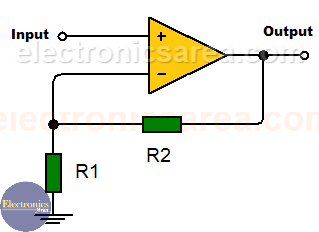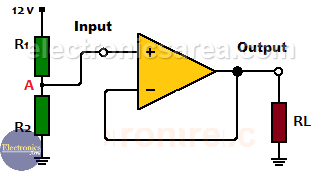# What is an Op Amp Voltage follower?

An Op Amp voltage follower (voltage follower using operational amplifier) is a circuit which has a voltage gain of 1. To better understand the operation of a voltage follower, we must remember the operation of an Op Amp  as a non-inverting amplifier. See the diagram below.

## Op Amp Non-inverting amplifier

The Op-Amp non-inverting amplifier gain is given by the formula: 1 + (R2/R1).If we make R2 resistor value equal to zero and make the R1 resistor value very large, we have an amplifier with gain G = 1. This means that the output signal has the same value as the input signal.  See the following diagram.

## Op Amp Voltage Follower (Voltage Buffer)

A buffer has an output that is exactly as the input. This behavior may initially seem useless, but has features that help solve impedance coupling problems.

• The input impedance of a buffer using an operational amplifier is very high, close to infinity
• The output impedance is very low, just a few ohms.If the input impedance is very high, it does not load the circuit that is sending the signal, and if its output impedance is low, it can deliver a sufficient amount of current to the circuit receiving the signal.

In other words a Buffer requests very little current from the circuit that gives the signal and greatly increases the capacity to deliver current to the circuit that receives the signal.

• Provides power gain and current gain. (voltage gain = 1).
• High input impedance. Op-amp takes no current from the input.

## Voltage follower applications

• Sample and hold circuits.
• Buffers for logic circuits.
• Active filters. Voltage followers can be used to isolate filter stages from each other, when building multistage filters.

## Op Amp voltage follower example

We need to get 6 volts from a 12 volt source to power a 100 ohm load resistor (RL).

We use two 100K resistors in series as a voltage divider (R1, R2). Since the resistors have the same value, the voltage between them is exactly 6 volts (A).If we connect the 100 ohm load to point A, the voltage will no longer be 6 volts, because the lower 100K resistor is in parallel with the load. The equivalent resistance of parallel resistors is: 100,000 x 100 / (100,000 + 100) = 99.9 ohms. We can safely assume the approximate value of 100 ohms.

Using the voltage divider again, the voltage at point A is: VA = 12 / (100K + 100) x 100 = 0.012 volts. Very different from the expected 6 volts.

To avoid this problem we use an op amp voltage follower. The non-inverting input of op amp is connected to A and the output is connected to the 100 ohm resistor (load). The load will have exactly 6 volts between its terminals.

•
•
•
•
•
•
•
•
•
•
•
•
•
•
•
•
•
•